# Riemann sphere

(diff) ← Older revision | Latest revision (diff) | Newer revision → (diff)

A sphere in the Euclidean space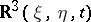onto which the extended complex plane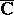is conformally and one-to-one transformed under stereographic projection. For example, the unit sphere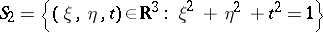can be taken as the Riemann sphere and the planecan be identified with the planesuch that the real axis coincides with the axisand the imaginary axis with the axis(see Fig.).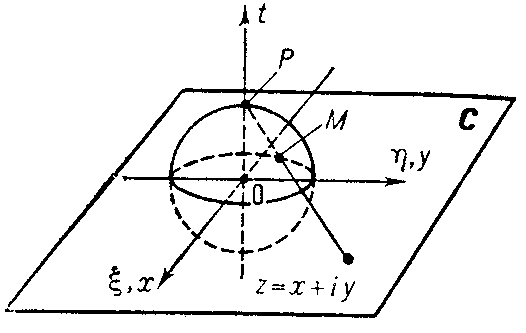Figure: r082010a

Under stereographic projection to each pointthere corresponds the point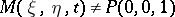obtained as the point of intersection of the ray drawn from the north pole of the sphere,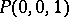, to the pointwith the sphere; the north polecorresponds to the point at infinity,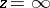. Analytically this relation can be expressed by the formulas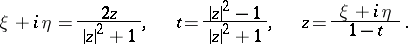(*)

In other words, the correspondence (*) determines a differentiable imbedding of the one-dimensional complex projective space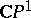into the space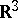in the form of the sphere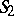. In many questions of the theory of functions, the extended complex plane is identified with the Riemann sphere. The exclusive role of the point at infinity of the planemay be dispensed with if the distance between two points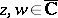is taken to be the chordal, or spherical, distance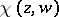between their images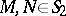: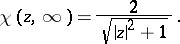A higher-dimensional complex projective space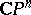,, can be imbedded into the spaceby a complex-dimensional stereographic projection, generalizing the formulas (*) (see ).

How to Cite This Entry:
Riemann sphere. Encyclopedia of Mathematics. URL: http://encyclopediaofmath.org/index.php?title=Riemann_sphere&oldid=18286
This article was adapted from an original article by E.D. Solomentsev (originator), which appeared in Encyclopedia of Mathematics - ISBN 1402006098. See original article